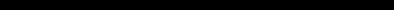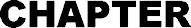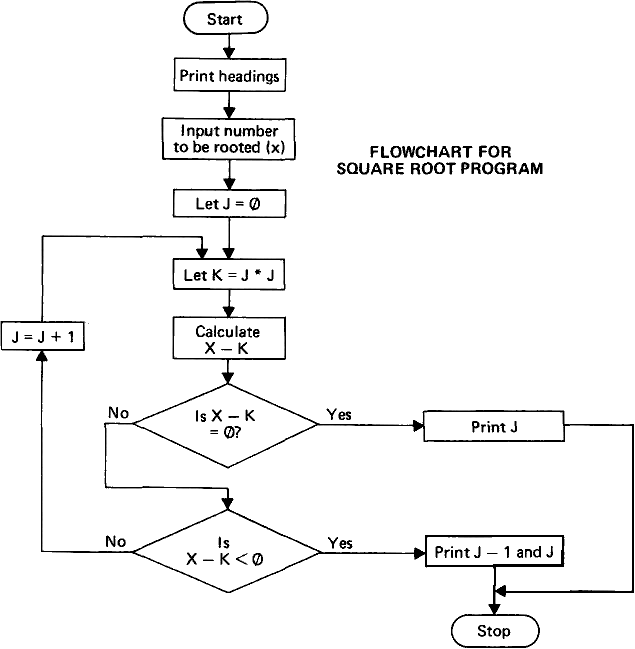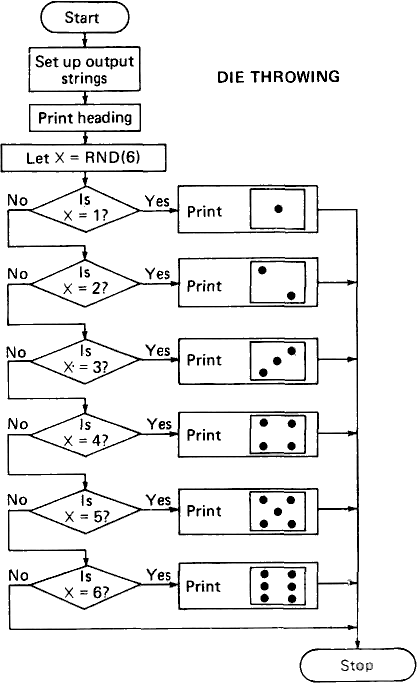# The ZX80 Operating ManualBRANCHING OUT Before we go on to real decisions lets have a look at the GO TO statement. This is of the form GO TO n where n is normally a line number. It can be a variable, though only the ZX-80 BASIC has the power to do this. When the ZX-80 comes to a GO TO statement it jumps straight to statement number n and executes that statement. If n is a variable  X, say  the computer will look at the value of X and jump to the statement with that number  assuming that there is. a statement with that number in your program. A further refinement of the GO TO statement is that n can be any integer expression such as A/10. Here again, this is possible with the ZX-80. Here is a program which illustrates the use of GOTO. 10 PRINT 'THIS IS STATEMENT 10" 20 GO TO 40 30 PRINT 'THIS IS STATEMENT 30" 40 PRINT "END OF PROGRAM" Now run the program. The computer responded with THIS IS STATEMENT 10 END OF PROGRAM It skipped statement 30. "That's not much use", you must be saying, "what's the point of - including a perfectly good statement which will never be executed?" OK Get back into command mode. Now, type GO TO 30, and then NEWLINE. The ZX-80 came back with THIS IS STATEMENT 30 END OF PROGRAM This demonstrates two uses of GO TO  first to jump unconditionally to an arbitrary statement (in this case statement 40); second as a command which causes the computer to jump to the desired statement (in this case 30) and start executing the program from there. THE IF STATEMENT This is the most powerful control statement in BASIC. It allows the user to incorporate decision-making into his programs. Here is a program for calculating square roots approximately. It does this by multiplying, a number by itself, starting with 0 and comparing the result with the number whose square root is to be found. If the product is less than the number to be rooted it increases the number, by 1 and tries again. 10 PRINT "SQUARE ROOT ROUTINE" 20 PRINT 'ENTER THE NUMBER TO BE ROOTED" 30 INPUT X 40 LET J=0 50 LET K = J*J 60 LET D = X-K 70 IF D=0 THEN GO TO 110     (Use SHIFT 3 to get THEN) 80 IF D<0 THEN GO TO 130     (< is SHIFT N) 90 LET J = J+1 100 GO TO 50 110 PRINT "THE ROOT IS" ; J 120 GO TO 140 130 PRINT " THE ROOT LIES BETWEEN (J-1); "AND ";J 140 STOP See the diagram on the next page. The form of the IF statement is: IF (CONDITION TO BE MET) THEN (DO THIS) 70 IF D=0 THEN GO TO 110 In line 70 the condition to be met was D = 0 and if this is so then J is exactly the square root of X, so we want to print THE ROOT IS J. In this case (DO THIS) is GO TO 110 which is the number of the PRINT statement we want. In most BASIC'S the only thing you can do in a IF statement is to GO TO a line number. The ZX-80 lets you do other things as well. In fact it lets you do almost anything! Some examples: 70 IF D=0 THEN PRINT "NUTS" 70 IF D=0 THEN INPUT G 70 IF D=0 THEN LET A = 10Any keyword can follow THEN in an IF statement  even things like LIST or RUN. The results can be rather peculiar if you use commands such as LIST. The examples listed above can be useful, though. The condition to be met is (in its simplest version) of the form: (Expression) (relational operator) (expression) This sounds rather a mouthful! A relational operator can be: =     equal to <     less than >     greater than Examples A = 2             A equal to 2 A < 10           A less than 10 A+B>C+D     A+B greater than C+D In these cases. A, 2, 10, A+B and C+D are the expressions. The expressions need not be a simple integer or integer variable. The statement     IF A = Z - (Z/B) * B THEN GO TO 10                                         ?expression is quite valid, and so is IF A + B > C+D THEN GO TO 20 The condition to be met can be even more complex because logical operators can be used. For example 10 IF NOT A =2 THEN GOTO 100            ?logical operator (use SHIFT 1 for NOT) This NOT operator negates the succeeding condition, so that the ZX-80 will jump to 100 if A does not equal 2. This program illustrates the use of the AND operator. 10 INPUT A 20 INPUT B 30 INPUT C 40 IF A = 1 AND B = 1 AND C = 1 THEN PRINT "OK"                     ?              ?Another logical operator                     ? (Use SHIFT 2 for AND) The condition to be met is that ALL three variables must be 1; if this is so it will print OK You can chain together as many conditions as you like in this way. Try running this program and see if you can make it print OK in any way other than by entering three 1's. The other logical operator is the OR operator. Edit the program by changing the AND's to OR's (OR is SHIFT B). When you run the program now you will find that it prints OK as long as one of the numbers entered is a 1. You can use brackets to group things together. Consider the following statements: (a)     40 IF A = 1 OR B = 1 AND C = 1 THEN PRINT "OK" (b)     40 IF (A = 1 OR B= 1) AND C = 1 THEN PRINT "OK" If you remember, in chapter 6 we said that some operators had priority over others  multiplications were carried out before divisions or additions, for instance. The same applies to logical operators. Their priority (in descending order) is NOT AND OR Thus, in (a) B = 1 AND C = 1 is taken first, so that the computer will print OK if B AND C are 1 but will also print OK if A =1. The brackets in (b) work in the same way as they did for arithmetic operators. Thus the ZX-80 will print OK if C = 1 and either B = 1 or A = 1. The operators NOT, AND and OR also allow you to produce conditional expressions. For example, if you require X=3 if A>B X = Q+R if A=B X = P if A < B The obvious way is: 110 IF A > B THEN LET X = 3 120 IF A = B THEN LET X = Q+R 130 IF A < B THEN LET X = P However you could instead write: 110 LET X = A > B - AND 3 OR A = B AND Q + R OR A < B AND P To round off this chapter on branching, here is a program which throws a die. The program uses a useful facility, the function RND (X) This generates a random number in the range 1 to X. It occurs in line 120 of the program  in this case X = 6 10 PRINT "DIE THROWING" 20 LET A\$ ="?   ?"  ? is SHIFT A 30 LET B\$= "  ?  " 40 LET C\$= "?    " 50 LET D\$ =    ? 60 LET E\$ ="....." 120 LET X = RND(6) 130 PRINT "YOU THREW..." 140 IF X = 1 THEN GO TO 200 150 IF X = 2 THEN GO TO 300 160 IF X = 3 THEN GO TO 400 170 IF X = 4 THEN GO TO 500 180 IF X = 5 THEN GO TO 600 190 IF X = 6 THEN GO TO 700 195 GO TO 1000 200 PRINT E\$ 205 PRINT E\$ 210 PRINT B\$ 215 PRINT E\$ 220 PRINT E\$ 230 GO TO 1000 300 PRINT C\$ 305 PRINT E\$ 310 PRINT E\$ 315 PRINT E\$ 320 PRINT D\$ 330 GO TO 1000 400 PRINT D\$ 405 PRINT E\$ 410 PRINT B\$ 415 PRINT E\$ 420 PRINT C\$ 430 GO TO 1000 500 PRINT A\$ 505 PRINT E\$ 510 PRINT E\$ 515 PRINT E\$ 520 PRINT A\$ 530 GO TO 1000 600 PRINT A\$ 605 PRINT E\$ 610 PRINT B\$ 615 PRINT E\$ 620 PRINT A\$ 630 GO TO 1000 700 PRINT A\$ 705 PRINT E\$ 710 PRINT A\$ 715 PRINT E\$ 720 PRINT A\$ 1000 STOP It's a bit of a strain to enter this program but it's quite fun to run. Note that lines 140 to 190 could have been replaced by 140 GOTO (X+1) * 100 If you want to make the program restart itself try editing the program: 1000 PRINT "HIT NEWLINE TO THROW AGAIN" 1100 INPUT X\$ 1200 CLS     (CLS is on key C) 1300 IF X\$ = "" THEN GOTO 120Two points: CLS clears the screen. If you didn't put this in the screen wouid fill up after 3 goes and the program would stop, giving an error message such as 5/305 (run out of screen at line 305). Secondly, the use of a INPUT statement to halt program execution is a useful trick. The string variable X\$ is used in statement 1300 when it is tested to see that only NEWLINE has been pressed; any other entry will stop the program (There is nothing between the quotation marks in 1300.) The reason that there is a IF statement at 1300 rather than a GO TO statement is that, while the ZX-80 is waiting for a string variable to be entered, the BREAK key (SHIFT SPACE) doesn't work. As a result it is difficult to get out of the program except by entering a very long string variable, a variable so long, in fact, that it makes the computer run out of storage space. This is very tedious, so it's better to use the IF statement. Then if you enter any character the program will stop. (If you do ever get into a situation when nothing you do seems to have any useful effect on the computer, try the BREAK key first. If this has no effect, give in and switch the ZX-80 off for a few seconds. You lose the current program, but this may be inevitable if you really have got yourself into a fix.)